Next: FastICA as EM-Algorithm with Up: Fast Algorithms for Bayesian Previous: EM-algorithm for Independent Component

# Fast EM-algorithm by Filtering of Gaussian Noise

With low noise variance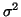the convergence of the EM-algorithm to the optimal value takes a time proportional to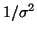. We will next show how the re-estimation step can be modified so that the convergence rate will be independent ofwhich yields a significant speedup ifis small.

Consider the case that we estimate the sources one at a time and that the sources are assumed to be whitened and the mixing matrix A orthonormal. Denote one of the source signals in the optimal solution as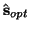. By optimality we mean that the standard EM-algorithm will eventually converge to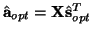with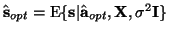.

When we have not yet found the optimal vector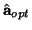, we have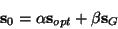where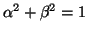. The noise sG is mostly due to the other sources and to a small extent the Gaussian noise in the data. We can think that the E-step filters away the noise by making use of the knowledge about the prior distribution of s. This gives one point of view into the slow convergence: in low noise case most of the unwanted signal sG is due to other sources and a slow convergence results. From this point of view, it is obvious that we can speed up the convergence if we can filter away also that part of sG which is due to other sources.

When we are far from the optimal solution, it is natural to assume that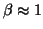and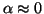. Since aand s0 are linearly related, we get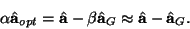If we can compute aG, we can adjust the vector ato take into account the apparent noise due to other sources. By the central limit theorem, the distribution of the sum of contributions from several other sources approaches Gaussian as the number of other sources increases. This leads to the following modification: we may estimate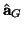using the same re-estimation whose result will be approximately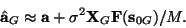where XG is the set of mixtures replaced by Gaussian noise with the same covariance as X and s0G is the source obtained as aTXG. The Gaussian source s0G is the projection of Gaussian noise to the subspace spanned by a and therefore represents the contribution of the other sources and some Gaussian noise to the estimated source s0. As derived above, we can eliminate much of this noise by updating a using the differencewhich is then normalized. The normalization can be done, since scaling of the sources is an undeterminacy in ICA.

Taking the difference yields approximately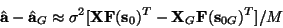which shows that the normalization cancels the effect offrom the learning rule: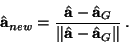We assumed above that there was a lot of Gaussian noise by approximating. It turns out that the above modification does not affect the optimal solutions of the algorithm, i.e., ifis a fixed point of the original EM-algorithm, it is also a fixed point of the modified algorithm. This follows immediately from the fact thatis always parallel to a since XG is spherically symmetric. To get a rough idea about why this is so, suppose there is a vector b which is orthogonal to a, i.e., bT a = 0. Then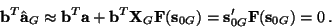The last step follows from the fact that s0G' is a projection to an orthogonal direction form s0G and by Gaussianity of XG, statistically independent form
F(s0G). But since this must hold for all b which are orthogonal to a, it follows thathas to be parallel to a.

In the next section we add validity to the result by showing that FastICA algorithm follows from this procedure.Next: FastICA as EM-Algorithm with Up: Fast Algorithms for Bayesian Previous: EM-algorithm for Independent Component
Harri Lappalainen
2000-03-09